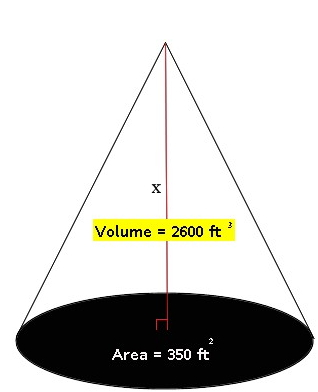Volume of Prisms, Cylinders, Cones, and Pyramids

Volume of Prisms, Cylinders,

Cones, and Pyramids

Volume is the quantity of space, calculated in cubic units, which a body occupies. The following lesson will explain the general formulas used to find the volume of the most frequently used solid figures: prisms, cylinders, pyramids, and cones.

Volume of Prisms

The volume of a prism is the product of the area of the base and the height of the object: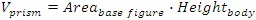For example, the volume of a cube is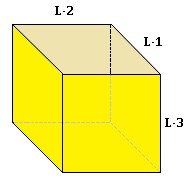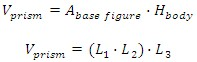In a cube all the sides have the same length (L1 = L2 = L3), therefore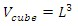Example

The box shown is 10 cm wide, 4 cm long, and 6 cm high. If you have gifts that are cubes, with a length of 1 cm, how many cubes will fit inside the box? What is the volume of the box?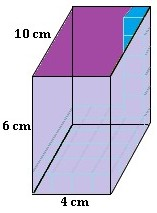The base of the box is 4 cm by 10 cm. The face is a rectangle, and to find the area of a rectangle we have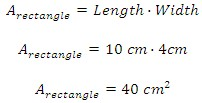Since we know that our gifts are cubes of length 1 cm, we can find their volume as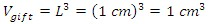with a base area of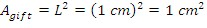If we take the proportion of the areas of the base of our box and the gifts we can find how many cubes we can fit in the base area of the box: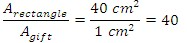So we can fit 40 of the gift boxes per base area, but we need to find out how many gifts will fit in the whole box. Five more layers of cubes can be placed on top of the base layer to fill the box. Now that the box contains 6 layers of gifts, with 40 gifts on each layer, the box will be filled by a total of 240 gifts. For this example, we used the method for finding the volume of a prism to find how many 1 cm3 gift boxes can fit.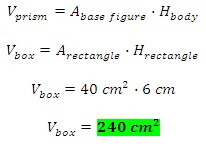Volume of a Cylinder

The volume of a cylinder follows the same logic as the prism.  The only difference is a change in the base, which is now a circle. Then the volume of the cylinder is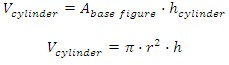Example

Find the volume of the following right circular cylinder.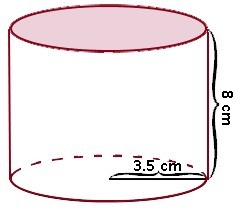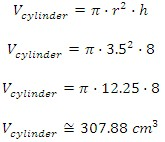Volume of a Pyramid

As we already saw, the volume of a prism is the area of the base times the height of the prism. The volume of the pyramid has the same base area and height as the prism, but with less volume than the prism. The volume of the pyramid is one third the volume of the prism.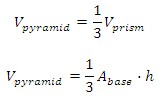Volume of a Cone

To find the volume of a cone, we follow the same principle as for the cylinder.  So we use the same method, but the base is now circular. Then we have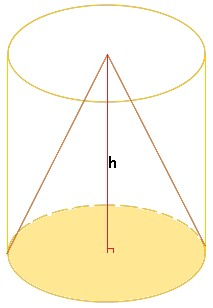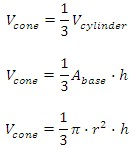For the following bodies, find the value for x.  Round to the nearest tenth when necessary.

1.)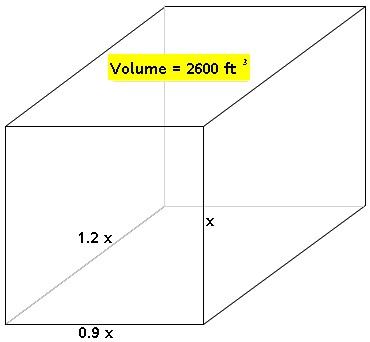2.)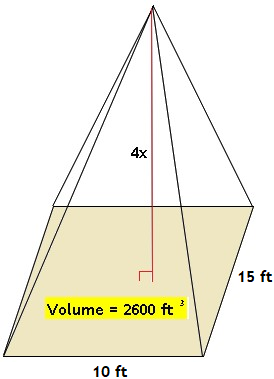3.)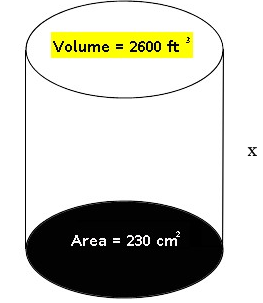4.)